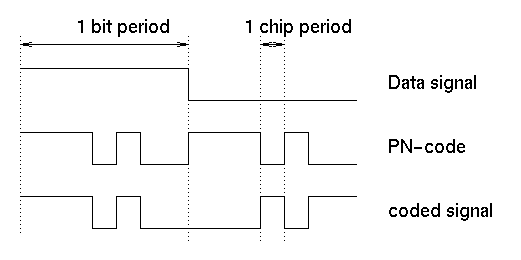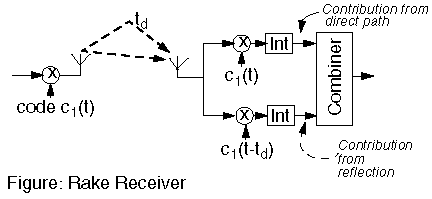JPL's Wireless Communication Reference Website

Chapter: Analog and Digital Transmission Section: CDMADirect Sequence CDMA

In Direct Sequence spread spectrum transmission, the user data signal is multiplied by a code sequence. Mostly, binary sequences are used. The duration of an element in the code is called the "chip time". The ratio between the user symbol time and the chip time is called the spread factor. The transmit signal occupies a bandwidth that equals the spread factor times the bandwidth of the user data.Figure: A DS-CDMA signal is generated by multiplication of a user data signal by a code sequence.
Source: Jack Glas, T.U. Delft.

In the receiver, the received signal is again multiplied by the same (synchronized) code. This operation removes the code, so we recover the transmitted user data.Different CDMA users use different codes. In this example the receiver sees the signal from user 1, while the signal from user 2 is heavily attenuated by the correlator (multiplier and integrator) in the receiver.

A CDMA receiver can retrieve the wanted signal by multiplying the receive signal with the same code as the one used during transmission. We find:where c1 is the code sequence used by user 1, Tc is the chip duration, td is a common time offset, shared between transmitter and receiver and N is the length of the code sequence.. Note that the receive code must be perfectly time aligned with the transmit code.

Popular code sequences are

• Maximum length or Pseudo Noise (PN) sequences.
• Gold codes, and
• Kasami codes.Figure: DS-CDMA signal with a jamming signal
Source: Jack Glas, T.U. Delft.

As despreading is the same operation as spreading a possible narrowband jammer signal in the radio channel is spread before the detector. Thus, the jam signal is attenuated by the spread factor ("spreading gain").

Near-Far Effect

A major difficulty in Direct Sequence transmission is the Near-Far effect. If more than one user is active, the incoming interference power is suppressed by the cross correlation between the code of the reference user and the code of the interferer. In the event that the interferer is closer to the receiver then the reference user, the interference components may not be sufficiently attenuated by the despreading process. In cellular CDMA systems, (adaptive) power control is needed to avoid this problem

Inherent Diversity

In a multipath fading channel, delayed reflections interfere with the direct signal. However, a DS-CDMA signal suffering from multipath dispersion can be detected by a rake receiver. This receiver optimally combines signals received over multiple paths.Different reflected waves arrive with different delays. A rake receiver can detect these different signals separately. These signals are then combined, using the diversity technique called maximum ratio combining.
Multipath self-interference is attenuated, because one can choose codes such thatCellular CDMA

IS95 is cellular telephone system that exploits advantages of DS-CDMA.